# 专栏 | 基于 Jupyter 的特征工程手册：特征选择（二）

https://github.com/YC-Coder-Chen/feature-engineering-handbook

# 目录：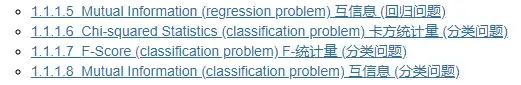#### 1.1.1.5 Mutual Information (regression problem) 互信息 (回归问题)

• 若两个变量均为离散变量: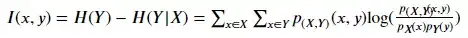p( , )( , ) 为x和y的联合概率质量函数 (PMF)， p ( )则为x的联合概率质量函数 (PMF)。

• 若两个变量均为连续变量: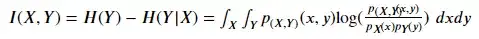p( , )( , ) 为x和y的联合概率密度函数 (PDF)，p ( )则为x的概率密度函数 (PDF)。连续变量情形下，在实际操作中，往往先对数据离散化分桶，然后逐个桶进行计算。

 A. Kraskov, H. Stogbauer and P. Grassberger, “Estimating mutual information”. Phys. Rev. E 69, 2004.

 B. C. Ross “Mutual Information between Discrete and Continuous Data Sets”. PLoS ONE 9(2), 2014.

import numpy as np
from sklearn.feature_selection import mutual_info_regression
from sklearn.feature_selection import SelectKBest

# 直接载入数据集
from sklearn.datasets import fetch_california_housing
dataset = fetch_california_housing()
X, y = dataset.data, dataset.target # 利用 california_housing 数据集来演示
# 此数据集中，X，y均为连续变量，故此满足使用MI的条件

# 选择前15000个观测点作为训练集
# 剩下的作为测试集
train_set = X[0:15000,:].astype(float)
test_set = X[15000:,].astype(float)
train_y = y[0:15000].astype(float)

# KNN中的临近数是一个非常重要的参数
# 故我们重写了一个新的MI计算方程更好的来控制这一参数
def udf_MI(X, y):
result = mutual_info_regression(X, y, n_neighbors = 5) # 用户可以输入想要的临近数
return result

# SelectKBest 将会基于一个判别方程自动选择得分高的变量
# 这里的判别方程为F统计量
selector = SelectKBest(udf_MI, k=2) # k => 我们想要选择的变量数
selector.fit(train_set, train_y) # 在训练集上训练
transformed_train = selector.transform(train_set) # 转换训练集
transformed_train.shape #(15000, 2), 其选择了第一个及第八个变量
assert np.array_equal(transformed_train, train_set[:,[0,7]])

transformed_test = selector.transform(test_set) # 转换测试集
assert np.array_equal(transformed_test, test_set[:,[0,7]]);
# 可见对于测试集，其依然选择了第一个及第八个变量

# 验算上述结果
for idx in range(train_set.shape):
score = mutual_info_regression(train_set[:,idx].reshape(-1,1), train_y, n_neighbors = 5)
print(f"第{idx + 1}个变量与因变量的互信息为{round(score,2)}")
# 故应选择第一个及第八个变量


#### 1.1.1.6 Chi-squared Statistics (classification problem) 卡方统计量 (分类问题)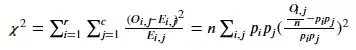# 首先，随机生成一个数据集
import pandas as pd
sample_dict = {'Type': ['J','J','J',
'B','B','B',
'C','C','C','C','C'],
'Output': [0, 1, 0,
2, 0, 1,
0, 0, 1, 2, 2,]}
sample_raw = pd.DataFrame(sample_dict)
sample_raw #原始数据，Output是我们的目标变量，Type为类别变量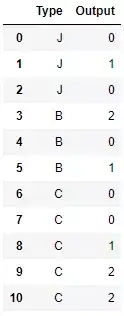# 下面利用独热编码生成布尔变量，并利用sklearn计算每一个布尔变量的chi2值
sample = pd.get_dummies(sample_raw)
from sklearn.feature_selection import chi2
chi2(sample.values[:,[1,2,3]],sample.values[:,])
# 第一行为每一个布尔变量的chi2值


(array([0.17777778, 0.42666667, 1.15555556]),
array([0.91494723, 0.8078868 , 0.56114397]))

# 下面直接计算原始变量Type与output统计学意义上的卡方统计量
# 首先，先统计每一个类别下出现的观测数，用于创建列联表
obs_df = sample_raw.groupby(['Type','Output']).size().reset_index()
obs_df.columns = ['Type','Output','Count']
obs_df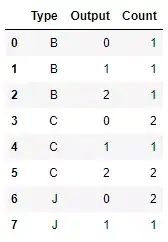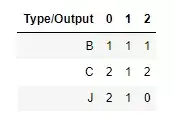from scipy.stats import chi2_contingency
obs = np.array([[1, 1, 1], [2, 1, 2],[2, 1, 0]])
chi2_contingency(obs) # 第一个值即为变量Type与output统计学意义上的卡方统计量


(1.7600000000000002,

0.779791873961373,

4,

array([[1.36363636, 0.81818182, 0.81818182], [2.27272727, 1.36363636, 1.36363636], [1.36363636, 0.81818182, 0.81818182]]))

# 而chi2方程算出来的布尔值之和为即为原始变量的统计意义上的卡方统计量
chi2(sample.values[:,[1,2,3]],sample.values[:,]).sum() == chi2_contingency(obs)


True

# 那么sklearn中的chi2是如何计算的呢？
# 不妨以第一个生成的布尔值为例，即Type为B
# chi2出来的值为0.17777778
# 而这与利用scipy以下代码计算出的计算一致
from scipy.stats import chisquare
f_exp = np.array([5/11, 3/11, 3/11]) * 3 # 预期频数为 output的先验概率 * Type为B 的样本数
chisquare([1,1,1], f_exp=f_exp) # [1,1,1] 即Type为B 的样本实际频数
# 即sklearn 中的chi2 仅考虑了Type为B情形下的列连表


Power_divergenceResult(statistic=0.17777777777777778,pvalue=0.9149472287300311)

import numpy as np
from sklearn.feature_selection import chi2
from sklearn.feature_selection import SelectKBest

# 直接载入数据集
from sklearn.datasets import load_iris # 利用iris数据作为演示数据集
X, y = iris.data, iris.target
# 此数据集中，X为连续变量，y为类别变量
# 不满足chi2的使用条件

# 将连续变量变为布尔值变量以满足chi2使用条件
# 不妨利用其是否大于均值来生成布尔值（仅作为演示用）
X = X > X.mean(0)

# iris 数据集使用前需要被打乱顺序
np.random.seed(1234)
idx = np.random.permutation(len(X))
X = X[idx]
y = y[idx]

# 选择前100个观测点作为训练集
# 剩下的作为测试集
train_set = X[0:100,:]
test_set = X[100:,]
train_y = y[0:100]

# sklearn 中直接提供了方程用于计算卡方统计量
# SelectKBest 将会基于一个判别方程自动选择得分高的变量
# 这里的判别方程为F统计量
selector = SelectKBest(chi2, k=2) # k => 我们想要选择的变量数
selector.fit(train_set, train_y) # 在训练集上训练
transformed_train = selector.transform(train_set) # 转换训练集
transformed_train.shape #(100, 2), 其选择了第三个及第四个变量
assert np.array_equal(transformed_train, train_set[:,[2,3]])

transformed_test = selector.transform(test_set) # 转换测试集
assert np.array_equal(transformed_test, test_set[:,[2,3]]);
# 可见对于测试集，其依然选择了第三个及第四个变量

# 验证上述结果
for idx in range(train_set.shape):
score, p_value = chi2(train_set[:,idx].reshape(-1,1), train_y)
print(f"第{idx + 1}个变量与因变量的卡方统计量为{round(score,2)}，p值为{round(p_value,3)}")
# 故应选择第三个及第四个变量


#### 1.1.1.7 F-Score (classification problem) F-统计量 (分类问题)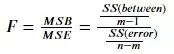import numpy as np
from sklearn.feature_selection import f_classif
from sklearn.feature_selection import SelectKBest

# 直接载入数据集
from sklearn.datasets import load_iris # 利用iris数据作为演示数据集
X, y = iris.data, iris.target
# 此数据集中，X为连续变量，y为类别变量
# 满足ANOVA-F的使用条件

# iris 数据集使用前需要被打乱顺序
np.random.seed(1234)
idx = np.random.permutation(len(X))
X = X[idx]
y = y[idx]

# 选择前100个观测点作为训练集
# 剩下的作为测试集
train_set = X[0:100,:]
test_set = X[100:,]
train_y = y[0:100]

# sklearn 中直接提供了方程用于计算ANOVA-F
# SelectKBest 将会基于一个判别方程自动选择得分高的变量
# 这里的判别方程为F统计量
selector = SelectKBest(f_classif, k=2) # k => 我们想要选择的变量数
selector.fit(train_set, train_y) # 在训练集上训练
transformed_train = selector.transform(train_set) # 转换训练集
transformed_train.shape #(100, 2), 其选择了第三个及第四个变量
assert np.array_equal(transformed_train, train_set[:,[2,3]])

transformed_test = selector.transform(test_set) # 转换测试集
assert np.array_equal(transformed_test, test_set[:,[2,3]]);
# 可见对于测试集，其依然选择了第三个及第四个变量

# 验证上述结果
for idx in range(train_set.shape):
score, p_value = f_classif(train_set[:,idx].reshape(-1,1), train_y)
print(f"第{idx + 1}个变量与因变量的ANOVA-F统计量为{round(score,2)}，p值为{round(p_value,3)}")
# 故应选择第三个及第四个变量


#### 1.1.1.7 Mutual Information (classification problem) 互信息 (分类问题)

【与1.1.1.5一样】互信息（Mutual Information）衡量变量间的相互依赖性。其本质为熵差，即 ( )− ( | )，即知道另一个变量信息后混乱的降低程度 。当且仅当两个随机变量独立时MI等于零。MI值越高，两变量之间的相关性则越强。与Pearson相关和F统计量相比，它还捕获了非线性关系。

• 若两个变量均为离散变量: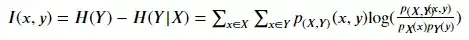p( , )( , ) 为x和y的联合概率质量函数 (PMF)， p ( )则为x的的联合概率质量函数 (PMF)。

• 若两个变量均为连续变量: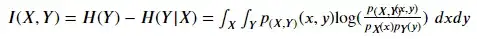p( , )( , ) 为x和y的联合概率密度函数 (PDF)，p ( )则为x的的联合概率密度函数 (PDF)。连续变量情形下，在实际操作中，往往先对数据离散化分桶，然后逐个桶进行计算。

 A. Kraskov, H. Stogbauer and P. Grassberger, “Estimating mutual information”. Phys. Rev. E 69, 2004.

 B. C. Ross “Mutual Information between Discrete and Continuous Data Sets”. PLoS ONE 9(2), 2014.

import numpy as np
from sklearn.feature_selection import mutual_info_classif
from sklearn.feature_selection import SelectKBest

# 直接载入数据集
from sklearn.datasets import load_iris # 利用iris数据作为演示数据集
X, y = iris.data, iris.target
# 此数据集中，X为连续变量，y为类别变量
# 满足MI的使用条件

# iris 数据集使用前需要被打乱顺序
np.random.seed(1234)
idx = np.random.permutation(len(X))
X = X[idx]
y = y[idx]

# 选择前100个观测点作为训练集
# 剩下的作为测试集
train_set = X[0:100,:]
test_set = X[100:,]
train_y = y[0:100]

# KNN中的临近数是一个非常重要的参数
# 故我们重写了一个新的MI计算方程更好的来控制这一参数
def udf_MI(X, y):
result = mutual_info_classif(X, y, n_neighbors = 5) # 用户可以输入想要的临近数
return result

# SelectKBest 将会基于一个判别方程自动选择得分高的变量
# 这里的判别方程为F统计量
selector = SelectKBest(udf_MI, k=2) # k => 我们想要选择的变量数
selector.fit(train_set, train_y) # 在训练集上训练
transformed_train = selector.transform(train_set) # 转换训练集
transformed_train.shape #(100, 2), 其选择了第三个及第四个变量
assert np.array_equal(transformed_train, train_set[:,[2,3]])

transformed_test = selector.transform(test_set) # 转换测试集
assert np.array_equal(transformed_test, test_set[:,[2,3]]);
# 可见对于测试集，其依然选择了第三个及第四个变量

# 验算上述结果
for idx in range(train_set.shape):
score = mutual_info_classif(train_set[:,idx].reshape(-1,1), train_y, n_neighbors = 5)
print(f"第{idx + 1}个变量与因变量的互信息为{round(score,2)}")
# 故应选择第三个及第四个变量### 觉得文章有用就打赏一下文章作者

#### 支付宝扫一扫打赏#### 微信扫一扫打赏